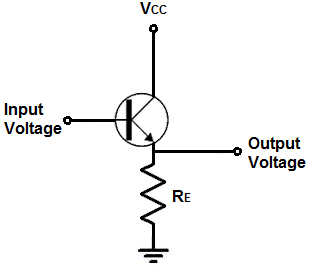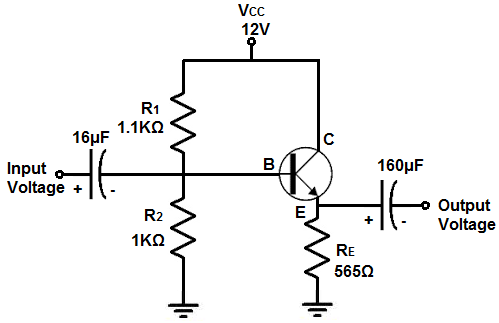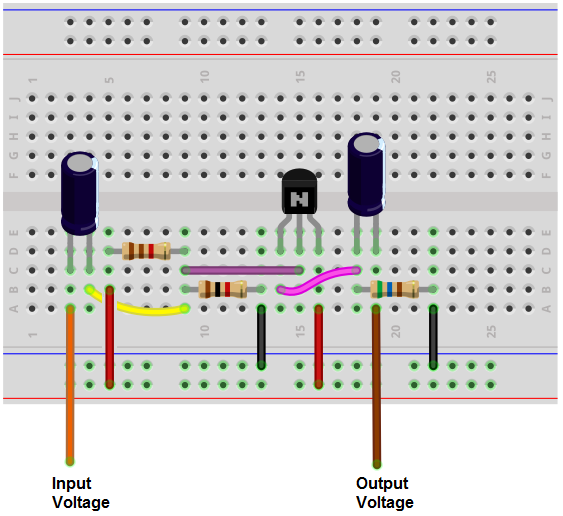﻿ How to Build an Emitter Follower Circuit

# How to Build an Emitter Follower CircuitIn this project, we will show how to build an emitter follower circuit.

An emitter follower circuit is a transistor circuit in which the voltage at the emitter follows the input voltage. It is a mirror image, so to speak, of the input voltage. Therefore, the voltage at the output is the same as the voltage at the input.

The emitter follower circuit is also called a common collector circuit, because the collector is common with the power supply voltage.

The point of an emitter follower circuit is that it can act as a buffer. Since it has a high input impedance and a low output impedance, it mimics the effect of a buffer.

Therefore, if a voltage line needs to be buffered it can be connected to the input of this circuit and the load that needs to be powered connects to the output of this circuit.

In our circuit, specifically, the input impedance will be over 50KΩ and the output impedance will be about 5Ω. This creates a pretty good buffer effect on any voltage that needs to be buffered.

In order to correctly design an emitter follower circuit, several factors must be taken into account, including how much DC voltage is supplied to the collector of the transistor, how much current is desired to be passed through the transistor, and the frequency cutoff of the AC signals entering the input. We will go over all these concepts in much depth below.

We will show all the components and facets into building an emitter follower circuit that functions very much like a buffer and can be used as a buffer.

This is great when you don't have a buffer chip available or a logic chip that you can convert into a buffer.

With just a single transistor and a few resistors and capacitors, you can have the buffer-like effect.

We will show in this circuit how to configure it and how to choose the values of the resistors and capacitors, which is called transistor biasing.

### Components Needed

• 2N3904 NPN transistor
• 1K‎Ω resistor
• 1.1K‎Ω resistor
• 500Ω resistor
• 16μF electrolytic capacitor
• 160μF electrolytic capacitor

The transistor that is chosen can really be any BJT NPN transistor. For this circuit, we choose the 2N3904 transistor.

For a lot of the values of the components we actually compute, you won't be able to find those precise values. For example, below we calculate one of the resistors to be 565Ω. This resistor does not exist. So we approximate. It's possible to find a 500Ω resistor and even if you can't you can put 2 1KΩ resistors in parallel to give an equivalent resistance of 500Ω.

A 1.1KΩ resistor also you will not find. So you can either approximate it down to 1KΩ or put a 1KΩ resistor in series with a 100Ω resistor. For all basic purposes, this is not necessary. You can simply connect a 1KΩ resistor instead of 1.1KΩ. It yields similar results.

The same approximations should be used for the capacitor values. Find as close of a value to the calculated capacitor values as possible and use those.

### Emitter Follower Circuit

The emitter follower circuit we will build with a single NPN BJT transistor is shown below.The breadboard circuit of the circuit above is shown below.So this is our circuit. We will now get into the details of why the various components in the circuit are needed and how to choose the values for them.

So the first thing is we decide how much voltage we're going to supply this circuit. This is the voltage, VCC, applied to the collector of the transistor. You can really choose any value from above VB, the base voltage, to the maximum value that the transistor can handle on the collector. This is typically 40-60V on most transistors. For our circuit, we will choose a reasonable voltage value of 12 for VCC. So this is the first consideration.

Next, now knowing VCC, we bias the base voltage of the circuit, which we means we select the voltage that we want to fall across the base of the transistor. What value do we want across the base? To answer this, we look at the complete picture. The amount of AC voltage that can be present at the output of the transistor is dependent on the DC voltage range that the circuit has. One on end of the spectrum, the transistor can swing up to 12V, which is VCC. On the other end of the spectrum, the transistor can swing down to near ground, about 0.7V. The reason the transistor cannot swing fully to ground is because the transistor will not turn on unless the diode is switched on and that happens at about 0.7V, the barrier voltage for the internal diode. Therefore, 0.7V is the floor of the circuit, and not ground or 0V. Therefore, with a power supply of 12V and a ground 0.7V, the transistor can swing from 0.7V up to 12V. Why this matters in regards to the voltage that we supply to the base is that whatever voltage comes into the circuit has a floor of 0.7V and a ceiling of 12V. Therefore, we want to bias the base voltage so that it falls in between these values. This gives a maximum peak-to-peak excursion for the signal and gives it the most room to operate without clipping. If the base voltage is biased incorrectly or well off the midway, the transistor can have significant clipping that produces poor output. For a 12V ceiling and 0.7V floor, the middle value would be (12 - 0.7V)/2 +0.7V= 6.35V. We have to take the diode voltage drop into consideration because the value at the base is 0.7V higher than at the output. By biasing the base voltage right in the middle of the ceiling and floor, it allows for maximum swing of the AC signal, called maximum peak-to-peak excursion. When biasing a transistor circuit that deals with AC signals, the AC signal that will can appear at the output without clipping is equivalent to the DC biasing. The AC signal will only be able to swing as far as the DC voltage biasing allows it. If the power supply is 20V and the floor is 0.7V, the transistor can swing from a peak value of 20V to a floor of 0.7V. Positioning the transistor at the midpoint of those voltages allows for the maximum swing of the AC signal from the ceiling to the floor. This is the optimum DC operating point for the circuit, called the Q-point or quiescent point. If you select a DC value too low, you will cause the signal to clip earlier at the bottom of the waveform. If you choose a DC operating voltage too high, you will cause the singal to clip at the top of the waveform.

So based on this point, we want the voltage at the base to be midway between 12V, the supply voltage, and 0.7V, which represents the floor. So that the VB= 0.7V + (12V-0.7V)/2, which is 6.35V. So in order to get this voltage, we have to choose our resistors that allows for this voltage to appear at the base. The voltage at the base is biased through 2 resistors. The resistors form a voltage divider and divide the supply voltage. We can divide our supply voltage into any voltage less than the power supply by choosing the correct value resistors. The base voltage, VB= VCC * (R2/R1+R2). In order to solve this equation, we choose the value of one resistor and then based on that resistor value compute the value of the other resistor based on this formula. So for the R2 resistor, a value of 1K‎Ω is chosen, as a reference point for the other resistor. Plugging these values into the formula, we get VB= VCC * (R2/R1+R2)= 6.35V (12V) (1KΩ). /(R1+1KΩ). Doing the math, this computes to a value of R1= 1.1KΩ. So in order to get a value of 6.35V at the base, the values of the resistors are R1 equal to 1.1KΩ and R2=1KΩ.

Next, we have to decide the value of the RE resistor. The value that we choose is integral so that we do not overheat and possibly destroy the transistor. How we choose RE is based on the value of the current, I E that we want following through the transistor and what is a safe current to flow through the transistor. So we'll just a choose a range that is a safe current that can flow through the transistor that won't burn it out. A safe current is 10mA. That is a decent amount of current and one that will not burn out the transistor. So the emitter resistor, RE= VE/IE, where VE is the voltage across the emitter and IE is the current flowing through the emitter. VE= VB-0.7V = 5.65V. The only difference in voltage between the base voltage and emitter voltage is the diode voltage drop between the 2 junctions. Because the transistor has a diode in it from the base to the emitter, the transistor has an internal voltage drop. Therefore, the emitter voltage is equal to the base voltage minus the voltage drop across the diode. So the formula, RE= VE/IE= 5.65V/10mA= 565‎Ω. So an RE resistor of about 500‎Ω would suffice well for this circuit.

Now lastly we have to choose the value of the capacitors in this circuit. Both capacitors have the same function. They allow only the AC signal to pass through and block the DC from passing through. So they function as coupling capacitors.

We'll first show how to calculate the value of the capacitor at the input.

In order to correctly choose an appropriate value of the capacitor, we have to take many things into account, which we will now discuss.

One, we have to decide the cutoff frequency of the circuit. This is the frequency that we want filtered out. If you're dealing with sound signals, the frequency range that is audible to humans is 20Hz to 20,000Hz. If you're building our transistor so that we only want audible signals passed through, 20Hz is a good cutoff frequency to choose. This means that any frequency at that range or lower will be filtered out to ground. So we will choose 20Hz as our cutoff frequency. If we take 20Hz and apply it to angular frequency, ω=2πf, we get ω=2πf≈125s-1. This is one part of the equation.

The next thing we must consider is the value of the resistance that the capacitor sees. The first part of this circuit from the capacitor and the resistor that it sees down to ground forms a high-pass filter, an RC high-pass filter. An RC high-pass filter is a circuit composed of a capacitor with a resistor connected to ground.

If you visualized current flowing into the circuit, current can go either 1 of 3 ways. Current can go through the capacitor and through the R2 resistor and down to ground. Current can go through the capacitor and through the R1 resistor. Or current can flow through the transistor and flow through the RE resistor down to ground. It may seem strange that current can flow through the R1 resistor but AC signals see DC voltage levels as ground as well. So even though it's at the DC level of 12V (through R1), to AC signals, it can also be a ground; this is why this can be a path down to ground as well.

So these are the 3 ways that current can flow in the circuit. EVen though current can take those paths, it's ultimately what a capacitor sees that determines the real resistance that it encounters. So we'll talk about this now. Resistors R1 and R2 are in parallel. Therefore, the capacitor sees the equivalent resistance of these 2 parallel resistors. So the capacitor sees the 1KΩ in parallel with the 1.1KΩ resistor. The equivalent resistance of these resistors is roughly 500Ω. So this the resistance that the capacitors sees at one pathway. The other pathway is when the current goes through the transistor and through resistor RE. This resistance will be βRE. With β equal to about 100, doing this math, this resistance computes to 56KΩ.

Remember that current always takes the path of least resistance. So between the options of 500Ω or 56KΩ, current will obviously take the 500Ω pathway.

So 500Ω is the equivalent resistance that the RC high-pass filter network sees and we know that we want a cutoff frequency of 20Hz. And calculating the angular frequency for 20Hz gives us, ω=2πf≈125s-1.

So going to the equation, REQC1= 1/125s-1= (500Ω)C1= 0.008s. Doing the math, this computes into a C1 value of 16µF. So the first capacitor is 16µF.

Now we move onto the second capacitor. And it's chosen similarly to the first capacitor.

We want the second capacitor to react similarly to the first, in regard to the frequency that we're dealing with. This is because the same frequency signal that is input is output. So you want to match the frequency response for both capacitors to the same frequency. So for the output capacitor, we also calculate it according to its 20Hz frequency response. Computing the angular frequency, we again get, ω=2πf≈125s-1.

We use the same formula again, REQC2= 1/125s-1.

What is REQ in this case? REQ is the resistance that the output capacitor sees in the circuit. Looking from the capacitor back to the input side, the capacitor sees a resistance of (R1 || R2)/β= 160 500Ω/100= 5Ω. So the output capacitor sees an equivalent resistance of 5Ω looking back to the input of the circuit.

So plugging these values into the equation, REQC2= 1/125s-1= (5Ω)C2= 0.008s. Solving for C2, we get 160µF. So this is the value of the output capacitor.

And now we've solved for all the values of the components in the circuit.

You can see based on the calculations that the circuit has a high input impedance and a low output impedance. As we calculated, the output impedance is βRE, which we calculated to be 56KΩ. The input impedance is (R1 || R2)/β= 5Ω. So you can see how this circuit has high input impedance and low output impedance.

So it functions very well as a buffer.

This circuit can be added to voltage line that needs to be buffered, which allows all the voltage to be transferred over, while giving out a low impedance output so that a load can be powered.

Know that this is a basic buffer circuit. It isn't as accurate as an actual buffer chip. For one, a buffer chip has greater impedance and lower output impedance. For another, it isn't a completely accurate voltage follower circuit. Being that the transistor has an internal diode that has a voltage drop roughly equivalent to 0.7V, it loses this voltage at the output. So the output signal is 0.7V lower than the input signal. So you can see it's not completely a voltage follower.

However, for all basic purposes, it functions very well as a low precision buffer.

Related Resources